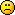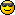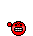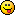# Math Is Fun Forum

Discussion about math, puzzles, games and fun.   Useful symbols: ÷ × ½ √ ∞ ≠ ≤ ≥ ≈ ⇒ ± ∈ Δ θ ∴ ∑ ∫  π  -¹ ² ³ °

You are not logged in.

## #1 Help Me ! » Probability and Statistics - Bayes' Theorem » 2010-10-01 15:36:01

mikau
Replies: 2

This problem is driving me crazy. I got part(a) and I really think I'm doing (b) correctly but I'm not getting the same answer as my book.

Componants of a certain type are shipped to a supplier in batches of ten. Suppose that 50% of all such batches contain no defective components, 30% contain one defective component and 20% contain two defective components. Two components from a batch are randomly selected and tested. What are the probabilities associated with 0,1 and 2 defective componants being in the batch under each of the following conditions:

(a) Neither tested component is defective
(b) One of the two tested components is defective

Here's how i solved (a)
Let Bk be the event that k defective components are in a batch, and let Tk be the event that k of the two tested components are defective.
We seek P(B0 | T0), P(B1 | T0), and P(B2 | T0)

First, observing that Bk are mutually exclusive and exhaustive, we may use Bayes' theorem and compute these as:
P(Bi | T0)=P(T0 | Bi) P(Bi) /[ P(T0 | B0)P(B0) +  P(T0 | B1)P(B1) + P(T0 | B2)P(B2)]

look at P(T0 | B0), P(T0 | B1) and P(T0 | B2).

P(T0 | B0) = 1, for if our batch contains no defective components, the probability of testing 0 defective components is 1.
P(T0 | B1) = (9 choose 2)/(10 choose 2), because we may test 2 out of all but the single defective component, and there are 10 choose 2 ways to test them total.
P(T0 | B2) = (8 choose 2)(10 choose 2), because we may test 2 out of all but the two defective components

Using these results in the formula for Bayes' theorem, gives me the correct answers for (a).

Now for (b), We seek P(B0 | T1), P(B1 | T1), and P(B2 | T1).
Using the same method as before, we now have to find the probabilities of P(T1 | B0), P(T1 | B1) and P(T1 | B2)
P(T1 | B0) = 0, for if there are no defective components, we cannot test 1 defective one
P(T1 | B1) = (9 choose 1)/(10 choose 2),  as there are (9 choose 1) ways to select the remaining non-defective component to be tested out of (10 choose 2) ways total
P(T1 | B2) = (8 choose 1)/(10 choose 2), as there are (8 choose 1) ways to select the remaining non-defective component.

Plugging all this in to bayes' theorem gives me:

P(B0 | T1) = 0
P(B1 | T1) = 0.628
P(B2 | T1) = 0.372

Seems absolutely sound to me. However, my book claims the answers are:
0
0.457
0.543

I am particularly frustrated since my reasoning seemed to work for (a)## #2 Re: This is Cool » "Factor Space" » 2010-01-27 18:52:55

that makes sense.

So what kind of useful invarient are we looking for in the context of this topic?

## #3 Re: This is Cool » "Factor Space" » 2010-01-27 17:37:19

i unfortunately haven't studied topology or group theory. I take mostly computer science courses these days. *sigh* i miss math!

## #4 Re: This is Cool » "Factor Space" » 2010-01-27 17:12:58

what exactly are you talking about when you say "invariants". I know the notion of invarients in programming/comp sci but thats about it.

## #5 Re: This is Cool » "Factor Space" » 2010-01-27 11:50:05

no i'm allowing powers to be rational (right?)

so I think that doesn't include transcendental numbers.

## #6 Re: This is Cool » "Factor Space" » 2010-01-27 11:44:50

this is a hasty response, but doesn't U include sqrt(2) = 2^(1/2), whereas K being rationals does not include sqrt(2)?

## #7 Re: Help Me ! » Distance of two points ! » 2010-01-27 11:34:05

I'm not sure I understand. Your first sentence makes no sense. Could you explain it a bit more carefully?

## #8 Re: This is Cool » "Factor Space" » 2010-01-27 10:05:18

I find this especially cool because it links linear algebra to number theory.

For instance! The fact that every number has a unique factorization follows from the fact that the primes form a basis of this vector space, and every element of a vector space can be expressed as a unique combination of its basis vectors.

Moreover, the scope of this goes beyond whole numbers because it includes numbers of the form (prime)^(rational).
So if I'm not mistaken, we can also use this to show that sqrt(2) =2^(1/2) is irrational. Because any fractional representation would be an attempt to create two distinct descriptions of sqrt(2) in terms of the basis vectors.

(edit) I take that back. I'm not sure you can prove the linear independence or primes without the FTA. Still I think the vector space notion is cool!

## #9 This is Cool » "Factor Space" » 2010-01-27 09:58:33

mikau
Replies: 10

I came up with this idea last night while brushing my teeth!

Let K denote the field of rationals.
Let U := { u | u = a[sub]1[/sub][sup]r1[/sup]a[sub]2[/sub][sup]r2[/sup]  a[sub]n[/sub][sup]rn[/sup]} where {a[sub]1[/sub]  a[sub]n[/sub]} are prime, and  {r[sub]1[/sub]  r[sub]n[/sub]} are in K
For all u,v in U and k in K:
let u+v := uv,
let ku := u[sup]k[/sup],
let 0 in U := 1
Then we have U is a vector space over K.

Proof:

Associativity:
(u+v)+w = (uv)w = u(vw) = u+(v+w)

Zero vector existence and properties:
0+u = (1)u = u = u(1) = u+0

Negative vector existence and properties:
For every vector u = a[sub]1[/sub][sup]r1[/sup]a[sub]2[/sub][sup]r2[/sup]  a[sub]n[/sub][sup]rn[/sup],  there exists (-u) = a[sub]1[/sub][sup]-r1[/sup]a[sub]2[/sub][sup]-r2[/sup]  a[sub]n[/sub][sup]-rn[/sup]  such that:
u+(-u) = (-u)+u = (u)(u[sup]-1[/sup]) = 1 = 0

Commutativity:
u+v = uv = vu = v+u

Distributivity of scalers:
k(u+v) = k(uv) = (uv)[sup]k[/sup] = u[sup]k[/sup]v[sup]k[/sup]= (u[sup]k[/sup])+(v[sup]k[/sup]) = ku + kv

Distributivity of vectors:
u(k1+k2)= u[sup](k1+k2)[/sup]=u[sup]k1[/sup]u[sup]k2[/sup]= u[sup]k1[/sup]+u[sup]k2[/sup]=k1u + k2u

Scaler Associativity:
(ab)u = u[sup](ab)[/sup]=u[sup](ba)[/sup]= (u[sup]b[/sup])[sup]a[/sup]=a(u[sup]b[/sup])=a(bu)

Unit Scaler:
(1)u = u[sup]1[/sup]=u## #10 This is Cool » implicit graph artwork » 2010-01-17 06:10:18

mikau
Replies: 1

I found this a while ago but I think I forgot to show the good folks at mathisfun.

I was totally blown away by this. Its an implict equation that draws a Star Wars Tie Fighter!

## #11 Re: Help Me ! » How is the conic section discriminant derived? » 2009-12-10 19:04:52

I don't know much about the significance of the descriminant in the context of the general conic equation, but its likely an advanced topic that you'd learn in a later course.  Right now, they're just telling you that B^2-4AC is defined to be the descriminant, you don't need to know where it came from right now. Often in math you learn how to use something before learning where it came from or why it works. And some things you can use without ever knowing exactly how they work. However! It is always good to ask questions and try to understand everything you can. :]

## #12 Re: This is Cool » intuitive way to calculate the sine and cosine of an angle » 2009-12-08 10:53:31

you said hello 3 times! ;]

Cordic method huh? I'll look it up

## #13 Re: This is Cool » intuitive way to calculate the sine and cosine of an angle » 2009-12-08 07:46:24

That works too! :]

Originally, I thought of it this way: start with the point P= (1,0) and reflect it about the line L through the origin and Q= (1,x/2^n), to produce a point R
now set P equal to Q, and Q equal to R, and repeat. In a way its kind of like rolling a cone of length 1 and circumference x/2^n, through 2^n revolutions until the large end falls onto the point (sin(x),cos(x))

## #14 Re: This is Cool » intuitive way to calculate the sine and cosine of an angle » 2009-12-08 07:13:54

Nice! And of course we can say a=x/2^M  for the general case! B)

(edit) also i think it should say that a[M]=sin(x) and b[M]=cos(x), otherwise the above difference equation just describes a sequence, and we don't know where to stop it.

## #15 This is Cool » intuitive way to calculate the sine and cosine of an angle » 2009-12-07 20:18:39

mikau
Replies: 7

Here is an amusing method for finding the sine of an angle that makes intuitive sense:

suppose we  want to find the sine of 1 radian, about 57 degrees.

First we choose a power of 2 that is large relative to the angle. I'll choose 32.

1/32 is fairly small and so sin(1/32)  ≈ 1/32, and cos(1/32)  ≈ 1.

Now that we know sin(1/32) and cos(1/32) we can use 5 applications of the double angle identities: sin(2x) = 2sin(x)cos(x) and cos(2x)=cos^2(x) - sin^2(x)  to get the final sine and cosine!
I worte a simple program to do it for me. Have a look:

sin(1/32): 0.03125 cos: 1
sin(2/32): 0.0625 cos: 0.999023
sin(4/32): 0.124878 cos: 0.994142
sin(8/32): 0.248293 cos: 0.972723
sin(16/32): 0.48304 cos: 0.884541
final (32/32): sin: 0.854537 cos: 0.549085

results by windows calculator:
sin: 0.8414709848078965066525023216303, cos: 0.54030230586813971740093660744298

Hey! Thats pretty close! Of course! you can make it more accurate by choosing a larger power of 2. Choosing 2^10 for instance yields: sin: 0.841881 cos: 0.540566

Its hardly the fastest way to do it, but its easy to understand why it works and I think that makes it fun!
Originally I envisioned this as a series of line reflections. But I eventually realized I was essentially using the double angle formulas. Maybe I'll post a diagram later.

## #16 Re: Dark Discussions at Cafe Infinity » Hello everyone! » 2009-12-07 19:03:21

thanks, Tigeree! :]

## #17 Re: Help Me ! » How is the conic section discriminant derived? » 2009-12-07 18:53:29

I don't see how Ax^2 + Bxy + Cy^2 can be turned into b^2-4ac.

Its easy! Watch, if we have 5x^2 + 3xy + 2y^2 and we want to find the discriminant, we observe A=5, B=3, and C=2, so to find B^2 -4*AC we just  substitute:
(3)^2 - 4*(5)*(2)= 9-40=-31.## #18 Re: Help Me ! » How to derive other equations from the general conic section equation? » 2009-12-07 18:45:31

Here's the generlal conic equation: Ax2 + Bxy + Cy2 + Dx + Ey + F = 0

Basically you make different shapes by assigning values to the coefficients A,B,C,D, E and F. Usually what you do is make some of those values 0 so the terms dissapear! For instance,
let A=1,B=0,C=1,D=0, E=0 and F=-1, then the above equation becomes

(1)x^2 + 0xy + (1)y^2 + (0)x + (0)y +(-1) = 0
this simplifies to:
x^2 + y^2 = 1 which is the equation of a circle!

Or if you let A = 1, E=-1 and everything else zero, you get:
(1)x^2 + 0xy + (1)y^2 + (0)x+(-1)y + (0) = 0
this simplifies to y=x^2 which is a parabola!

So its all just a question of what coefficients should be zero!## #19 Dark Discussions at Cafe Infinity » Hello everyone! » 2009-12-07 18:31:43

mikau
Replies: 7

I started avoiding these forums because one member was making things a bit unpleasant for me but it appears that member isn't posting so much anymore.

Anyways! I got my associates degree in computer science and i should have one in math but I was one humanties class shy!but I changed schools and I'm continuing my Computeer Science education though i'm considering switching my major to math. I am unhappy with how little mathematics I've learned since I entered college, relative to before when I was self taught,  and I'm trying to find time for more self study. These forums are also a great place to learn and practice math, by asking and answring questions, so I thought I should frequent them more!anyways, good to be back everyone!

## #20 Re: Dark Discussions at Cafe Infinity » How would you move Mnt. Fuji? » 2009-07-03 15:31:52

why is that, Simron? i don't use python..

## #21 Jokes » Random jokes » 2009-06-29 15:16:56

mikau
Replies: 0

Whats a mathematicians favorite soda?

square root beer!

Whats a mathematicians favorite beer?

Natural Lagger!

Why did einstein cross the road?

well he insists the road crossed him!

Why did the mathematician cross the road?

he never crossed it, he approached it asymptotically

what is a helicopter bagel?

its like a plain bagel only it takes off vertically!

:]

## #22 Re: Dark Discussions at Cafe Infinity » Tsk tsk for British parents » 2009-06-29 04:31:08

I can easily say that at certain points of my life I spent over 20 hours a month playing games, and for the most part don't regret a minute of it.

No offense Ricky but I almost want to laugh! That's what, like 40 minutes a day? My parents only wish I played that little when I was younger!If you consider that excessive than I'd say you have very good self control/disciplinewish i had that

## #23 Re: This is Cool » Code Breaking Challenge! » 2009-06-29 04:21:56

we could write an AI thread evaluator to determine the posters Anythony-R-Brownyness, on a scale of 0 to 100%, and anyone above 90% is automatically banned.

## #24 Re: Dark Discussions at Cafe Infinity » How would you move Mnt. Fuji? » 2009-06-27 20:12:10

hey! no delegation!## #25 Re: Dark Discussions at Cafe Infinity » How would you move Mnt. Fuji? » 2009-06-27 18:51:31

nice! but you might as well move a big rock or that center of mass is gonna move awfully slow!